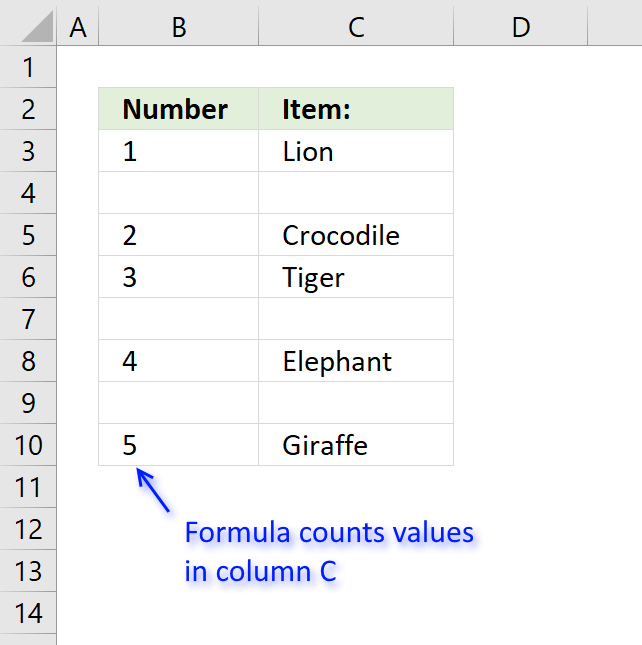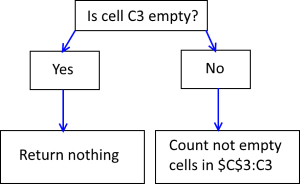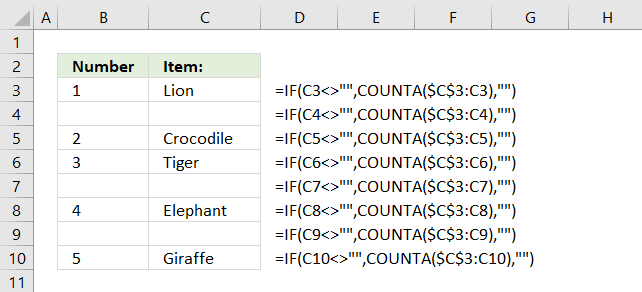Author: Oscar Cronquist Article last updated on November 16, 2018The formula in column B returns a running count based on values in column C.

Formula in cell B3:

=IF(C3<>"",COUNTA(\$C\$3:C3),"")

The formula consists of two Excel functions. The IF function checks if the corresponding value in column C is not empty.If cell C3 is not empty the COUNTA function counts the number of cells in cell range \$C\$3:C3 that are not empty.

Cell range \$C\$3:C3 has one cell that is not empty so the formula returns 1 in cell B3.

Note that cell reference \$C\$3:C3 expands when you copy cell B3 and paste to cells below.For example, in cell B4 the cell reference changes to \$C\$3:C4.

If cell C3 is empty the formula returns nothing.